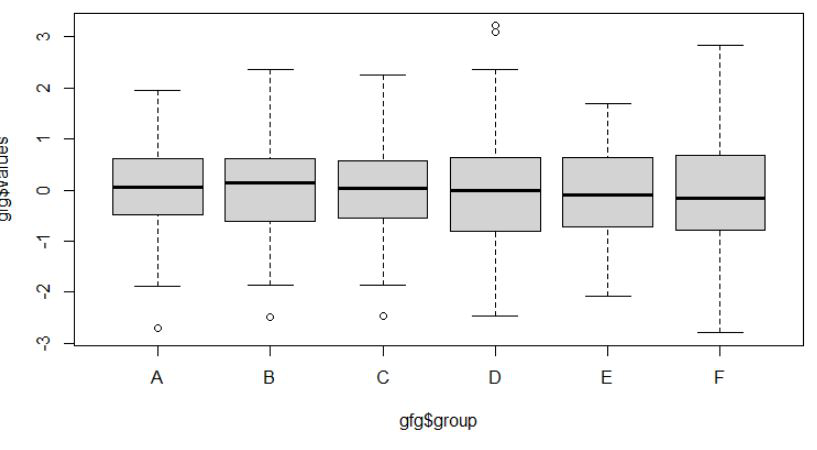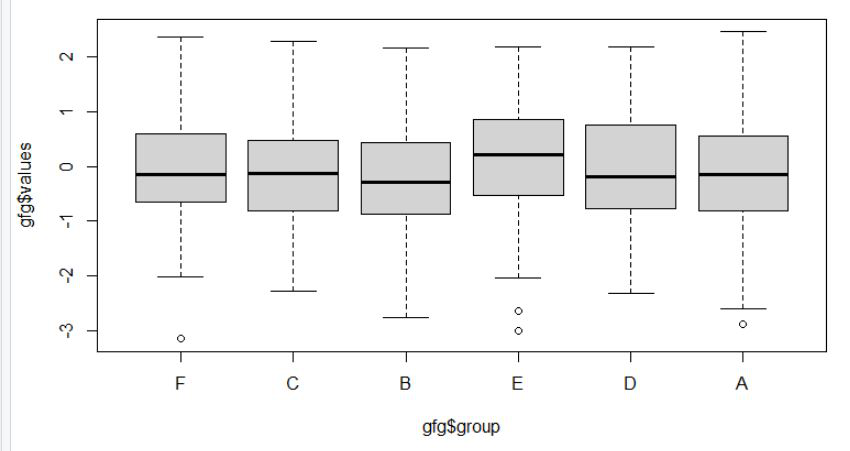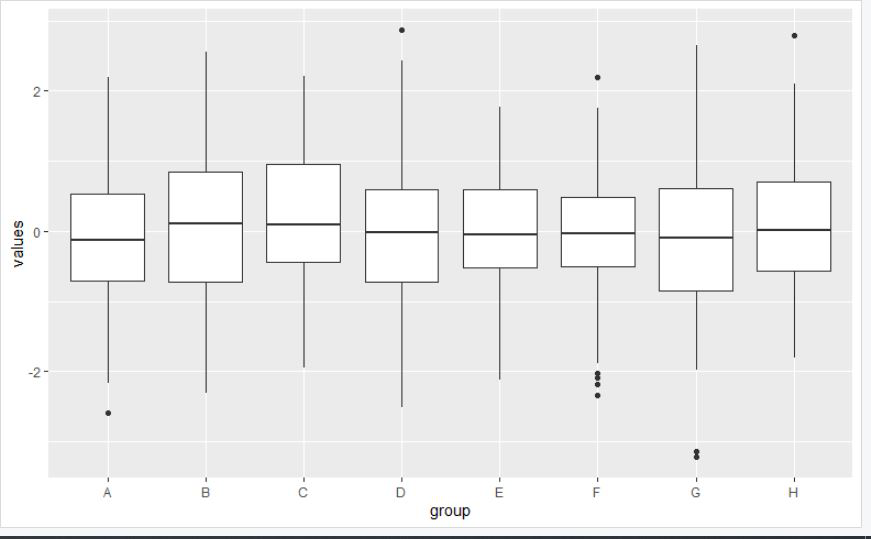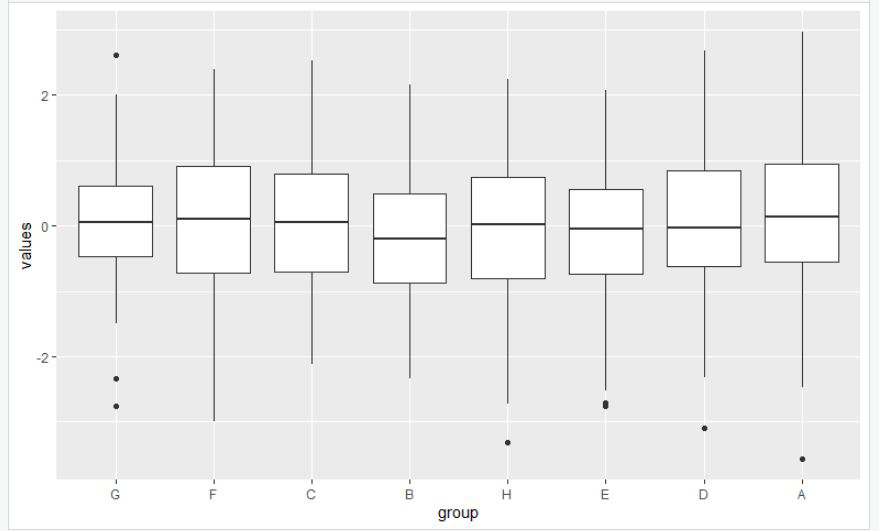GeeksforGeeks App
Open AppBrowser
Continue

## Related Articles

In this article, we will discuss how to reorder the boxplot in the R programming language.

## Reordering the boxplot manually using the factor function

Under this approach to reordering the boxplot, the user needs to call the factor function which is an inbuilt function of the R programming language, and then the user needs to pass the new order of the boxplot as per the requirement by the user in a vector as its parameters and further this process will be leading to the reordering of the boxplot as configured by the user in the R programming language.

Example 1:

In this example, we will be simply creating the boxplot of 6 different units each carrying 600 random data points with the help of the boxplot function and with the factor function we will be reordering the units in the R programming language

Initial boxplot without any modification:## R

 `# create a dataframe with letters and value``gfg < - ``data.frame``(group=``rep``(``c``(``'A'``, ``'B'``, ``'C'``, ``'D'``, ``'E'``, ``'F'``)),``                   ``values=``rnorm``(600))` `# factor the data``gfg\$group < - ``factor``(gfg\$group, ``c``(``"F"``, ``"C"``, ``"B"``, ``"E"``, ``"D"``, ``"A"``))` `# plot the data``boxplot``(gfg\$values ~ gfg\$group)`

Output:Example 2:

In this example, we will be simply creating the boxplot of 8 different units each carrying 800 random data points in the ggplot2 and with the factor function we will be reordering the units in the R programming language

Initial boxplot without any modification:Example:

## R

 `# load the library``library``(``"ggplot2"``)` `# get the data``gfg < - ``data.frame``(group=``rep``(``c``(``'A'``, ``'B'``, ``'C'``, ``'D'``, ``'E'``,``                               ``'F'``, ``'G'``, ``'H'``)), values=``rnorm``(800))``gfg\$group < - ``factor``(gfg\$group, ``c``(``"G"``, ``"F"``, ``"C"``, ``"B"``, ``"H"``, ``"E"``, ``"D"``, ``"A"``))` `# plot the data``ggplot``(gfg, ``aes``(group, values)) + ``geom_boxplot``()`

Output:My Personal Notes arrow_drop_up